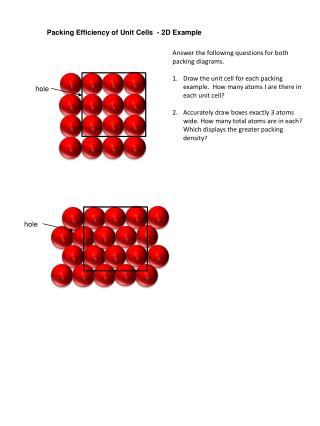DownloadDownload Presentationhole

# hole

Download Presentation## hole

- - - - - - - - - - - - - - - - - - - - - - - - - - - E N D - - - - - - - - - - - - - - - - - - - - - - - - - - -
##### Presentation Transcript

1. Packing Efficiency of Unit Cells - 2D Example Answer the following questions for both packing diagrams. Draw the unit cell for each packing example. How many atoms I are there in each unit cell? Accurately draw boxes exactly 3 atoms wide. How many total atoms are in each? Which displays the greater packing density? hole hole

2. Packing Efficiency of Unit Cells Assume atoms hard spheres r 2r SIMPLE CUBIC PACKING Top Side views 2r

3. 7 8 4 3 FACE CENTERED CUBIC - FCC 6 5 1 2 4r a a

4. FACE CENTERED CUBIC - FCC unit cell a 4r a √2 a a x2 = a2 + a2 x = √2 a

5. How many red spheres touch yellow in first layer A? A A How many red spheres touch yellow in first layer Aif there is an identicallayer A in front of and behind the first A? What is the coordination number for scp? unit cell: simple cubic

6. BODY CENTERED CUBIC PACKING - ABAB How many spheres touch yellow in layer B? A B How many spheres touch yellow in layer Bif a red layer A is added below and above B? What is the coordination number for bcc?

7. CUBIC CLOSEST PACKING How many spheres touch yellow in layer A? A How many spheres touch yellow in layer B? How many spheres touch yellow in layer C? What is the coordination number for FCC (or hcp)?